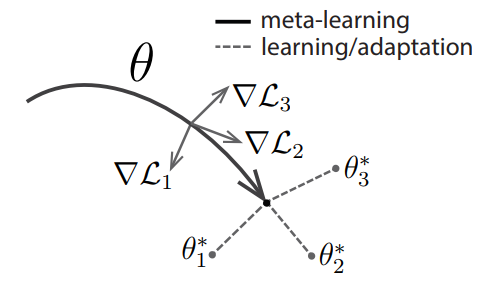# Model-Agnostic Meta-Learning¶

Model-Agnostic Meta-Learning (MAML)： 与模型无关的元学习，可兼容于任何一种采用梯度下降算法的模型。 MAML 通过少量的数据寻找一个合适的初始值范围，从而改变梯度下降的方向， 找到对任务更加敏感的初始参数， 使得模型能够在有限的数据集上快速拟合，并获得一个不错的效果。 该方法可以用于回归、分类以及强化学习。

## 1 MAML¶

MAML 是典型的双层优化结构，其内层和外层的优化方式如下：

### 1.1 MAML 内层优化方式¶

$\min _{\phi} L_{T_{i}}\left(f_{\phi}\right)$

$\theta_{i}^{N}=\theta_{i}^{N-1}-\alpha\left[\nabla_{\phi} L_{T_{i}}\left(f_{\phi}\right)\right]_{\phi=\theta_{i}^{N-1}}$

### 1.2 MAML 外层优化方式¶

$\min _{\theta} \sum_{T_{i}\sim p(T)} L_{T_{i}}\left(f_{\theta_{i}^{N}}\right)$

$\theta \leftarrow \theta-\beta \sum_{T_{i} \sim p(T)} \nabla_{\theta}\left[L_{T_{i}}\left(f_{\phi}\right)\right]_{\phi=\theta_{i}^{N}}$

## 2 MAML 算法流程¶

1. randomly initialize $$\theta$$

2. while not done do:

3. sample batch of tasks $$T_i \sim p(T)$$

4. for all $$T_i$$ do：

1. evaluate $$\nabla_{\phi}L_{T_{i}}\left(f_{\phi}\right)$$ with respect to K examples

2. compute adapted parameters with gradient descent: $$\theta_{i}^{N}=\theta_{i}^{N-1} -\alpha\left[\nabla_{\phi}L_{T_{i}}\left(f_{\phi}\right)\right]_{\phi=\theta_{i}^{N-1}}$$

5. end for

6. update $$\theta \leftarrow \theta-\beta \sum_{T_{i} \sim p(T)} \nabla_{\theta}\left[L_{T_{i}}\left(f_{\phi}\right)\right]_{\phi=\theta_{i}^{N}}$$

7. end while

MAML 中执行了两次梯度下降 (gradient by gradient)，分别作用在基学习器和元学习器上。图1给出了 MAML 中特定任务参数 $$\theta_{i}^{*}$$ 和元级参数 $$\theta$$ 的更新过程。## 3 MAML 模型结构¶

MAML 是一种与模型无关的元学习方法，可以适用于任何基于梯度优化的模型结构。

## 4 MAML 分类结果¶

Method

5-way 1-shot

5-way 5-shot

20-way 1-shot

20-way 5-shot

MANN, no conv (Santoro et al., 2016)

82.8 $$\%$$

94.9 $$\%$$

MAML, no conv

89.7 $$\pm$$ 1.1 $$\%$$

97.5 $$\pm$$ 0.6 $$\%$$

Siamese nets (Koch, 2015)

97.3 $$\%$$

98.4 $$\%$$

88.2 $$\%$$

97.0 $$\%$$

matching nets (Vinyals et al., 2016)

98.1 $$\%$$

98.9 $$\%$$

93.8 $$\%$$

98.5 $$\%$$

neural statistician (Edwards & Storkey, 2017)

98.1 $$\%$$

99.5 $$\%$$

93.2 $$\%$$

98.1 $$\%$$

memory mod. (Kaiser et al., 2017)

98.4 $$\%$$

99.6 $$\%$$

95.0 $$\%$$

98.6 $$\%$$

MAML

98.7 $$\pm$$ 0.4 $$\%$$

99.9 $$\pm$$ 0.1 $$\%$$

95.8 $$\pm$$ 0.3 $$\%$$

98.9 $$\pm$$ 0.2 $$\%$$

Method

5-way 1-shot

5-way 5-shot

fine-tuning baseline

28.86 $$\pm$$ 0.54 $$\%$$

49.79 $$\pm$$ 0.79 $$\%$$

nearest neighbor baseline

41.08 $$\pm$$ 0.70 $$\%$$

51.04 $$\pm$$ 0.65 $$\%$$

matching nets (Vinyals et al., 2016)

43.56 $$\pm$$ 0.84 $$\%$$

55.31 $$\pm$$ 0.73 $$\%$$

meta-learner LSTM (Ravi & Larochelle, 2017)

43.44 $$\pm$$ 0.77 $$\%$$

60.60 $$\pm$$ 0.71 $$\%$$

MAML, first order approx.

48.07 $$\pm$$ 1.75 $$\%$$

63.15 $$\pm$$ 0.91 $$\%$$

MAML

48.70 $$\pm$$ 1.84 $$\%$$

63.11 $$\pm$$ 0.92 $$\%$$

## 5 MAML 的优缺点¶

• 适用于任何基于梯度优化的模型结构。

• 双层优化结构，提升模型精度和泛化能力，避免过拟合。

• 存在二阶导数计算

## 6 对 MAML 的探讨¶

• 每个任务上的基学习器必须是一样的，对于差别很大的任务，最切合任务的基学习器可能会变化，那么就不能用 MAML 来解决这类问题。

• MAML 适用于所有基于随机梯度算法求解的基学习器，这意味着参数都是连续的，无法考虑离散的参数。对于差别较大的任务，往往需要更新网络结构。使用 MAML 无法完成这样的结构更新。

• MAML 使用的损失函数都是可求导的，这样才能使用随机梯度算法来快速优化求解，损失函数中不能有不可求导的奇异点，否则会导致优化求解不稳定。

• MAML 中考虑的新任务都是相似的任务，所以没有对任务进行分类，也没有计算任务之间的距离度量。对每一类任务单独更新其参数初始值，每一类任务的参数初始值不同，这些在 MAML 中都没有考虑。